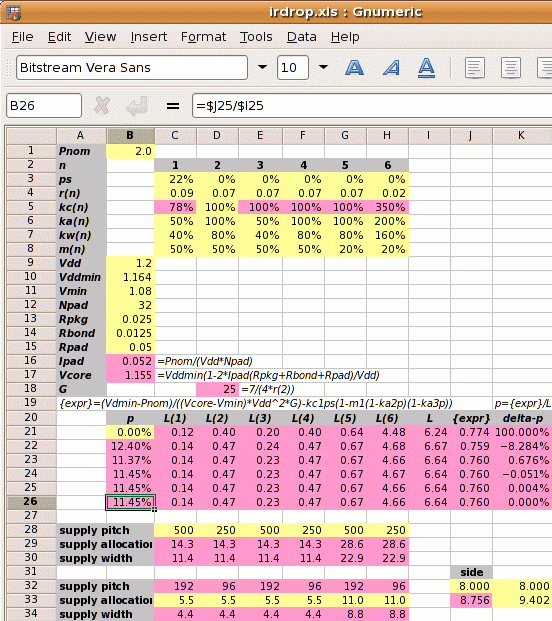In order to calculate the width of power straps in a design, firstly the design attributes need to be set out in a table like the one on the right.

Then the calculation proceeds in 5 steps.

Step 1: Calculate Ipad and Vcore:

Vcore =

Step 2: Calculate the reference power supply conductance G:

G =
 7 4×r2

Step 3: Set out the values of kan, kwn, kcn and mn for each metal layer, and use these to calculate the value of L.
(L is the parallel metal conductivity coefficient. That is, how much bigger the total metal conductivity will be than the reference metal conductivity due to multiple metal layers with different conductivities and widths. kan and kwn set out the relative allocations and widths we want for the power supply on each metal layer. kcn says how much more conductive a metal layer is than the value 1⁄r2 for metal-2.)

metal layer   1      2      3      4      5      6      7      8
kan
power metal allocated coefficient
kwn
power metal used coefficient
kcn
metal conductivity coefficient
mn
core area blocked

The expression for L is:

 L = kw1kc1(1-ps)(1-m1(1-ka2p)(1-ka3p))+ kw2kc2(1-m2(1-ka2p)(1-ka3p))+ … + kw8kc8(1-m8(1-ka2p)(1-ka3p))

The value of L is a function of p, the amount of metal allocated to the power supplies and whose value we do not know. Since we don't know it, we estimate it. Our first estimate for p will be p=0%.

Step 4: Calculate the power strap allocation percentage p.

 m1′ = m1×(1-ka2p)(1-ka3p)
p =
 { Vddmin×Pnom −kc1×ps(1-m1′) } × 1 (Vcore−Vmin)×Vdd2×G L

The first value of p uses the value of L calculated with p=0. This allows a better estimate of L and the iteration leads to the solution, as shown in the spreadsheet example on the right. The yellow boxes are user input like core power consumption Pnom or the initial estimate for p. The pink boxes are calculated values.

Step 5: Calculate the new core size x′.

 x′ = x √(((1−ka2p)(1−ka3p))

The value of p sets the new core size and the power strap allocation and pitch.
If we want to set the power straps to a width of Wsn, then their pitch is:

 horizontal pitch M1 = 2×Ws1 ⁄ (kw1×p) vertical pitch M2 = 2×Ws2 ⁄ (kw2×p) … vertical pitch M8 = 2×Ws8 ⁄ (kw8×p)

Design Attribute Value
Pnom core power consumption
ps fraction of metal-1 in the standard cells used for power supplies
rn resistivity of metal layer n in ohms per square
kan
 user defined   ratio of metal layer n allocated to power metal-2 allocated to power

kwn
 user defined   ratio of metal layer n used for power metal-2 allocated to power

mn percentage of metal layer n which is blocked
Vdd the nominal supply voltage
Vddmin the minimum supply voltage, typically 5% less than the nominal
Vmin the desired voltage at the centre of the die, typically 10% less than the nominal
Rpkg the resistance of the package leadframe
Rbond the resistance of the bond wire